Courses
Courses for Kids
Free study material
Offline Centres
More

# NCERT Solutions for Class 10 Maths Chapter 6 - ExerciseLast updated date: 01st Dec 2023
Total views: 530.7k
Views today: 12.30k

## NCERT Solutions for Class 10 Maths Chapter 6 Triangles (Ex 6.5) Exercise 6.5

Our team at Vedantu is always striving to create the most reliable and beneficial solutions for students and our NCERT Solutions Class 10 Maths Ex 6.5 is an example of the same. The way our subject matter experts have given Ex 6.5 Class 10 Maths NCERT Solutions, even those of you who find Mathematics difficult would start enjoying the subject. These accurate and error-free Maths Class 10 Chapter 6 Exercise 6.5 solutions are based on the latest CBSE curriculum so that you can expect high scores in maths by availing these solutions. Subjects like Science, Maths, English will become easy to study if you have access to NCERT Solution Class 10 Science, Maths solutions, and solutions of other subjects.

### Importance of NCERT Solutions for Class 10 Maths Chapter 6 Triangles (Ex 6.5) Exercise 6.5

Chapter 6 of the class 10 maths syllabus is on Triangles. Triangles is one of the most important chapters covered in class 10 that will be used throughout a student’s academic career in science and maths. This chapter deals with the important aspects of triangles that are covered in the expert-curated solutions on the topic. NCERT Solutions for Class 10 Maths Chapter 6 Triangles will help students get a good hold of the various types of problems that can be solved in their exams. The solutions also touch upon the important properties of triangles.

### Triangles - Important Properties

Like any other shape in Maths, triangles too have some properties that distinguish them from others. The following is a list of some important properties of triangles.

• All triangles have 3 sides and 3 angles.

• The 3 angles of a triangle add up to a total of 180 degrees.

• The sum of the exterior angles of a triangle is 360 degrees.

• The side that is opposite the smallest interior angle is always the shortest. Similarly, the side opposite the largest interior angle is always the largest.

• Consecutive interior and exterior angles are supplementary.

• The length of the third side of any triangle is always smaller than the sum of the lengths of the other two sides. Likewise, the length of the third side of a triangle is always greater than the difference between the lengths of the other two sides.

Watch videos on
NCERT Solutions for Class 10 Maths Chapter 6 - ExerciseTRIANGLES Class 10 in One Shot (Complete Chapter) | CBSE 10 Maths Chapter 6 - Term 1 Exam | Vedantu
Vedantu 9&10
SubscribeShare
8K likes
132.1K Views
2 years agoTRIANGLES L-1 (Similarity and Criteria for Similarity) CBSE Class 10 Math Chapter 6 | 1st Term Exam
Vedantu 9&10
6.4K likes
161.7K Views
2 years agoTriangles in One Shot | CBSE Class 10 Maths Chapter 6 | NCERT Solutions | Vedantu Class 9 and 10
Vedantu 9&10
10.7K likes
265.2K Views
3 years agoTriangles L1 | Basic Proportionality Theorem (Thale's Theorem) | CBSE Class 10 Maths NCERT | Vedantu
Vedantu 9&10
9.8K likes
298.7K Views
3 years ago
See More## Access NCERT Solutions for Class 10 Maths Chapter - 6 - Triangles

EXERCISE NO: 6.5

1. Sides of triangles are given below. Determine which of them are right triangles? In case of a right triangle, write the length of its hypotenuse.

(i) $\mathbf{7}$ cm, $\mathbf{24}$ cm, $\mathbf{25}$ cm.

Ans: We are provided that the sides of the triangle are of lengths $7$ cm, $24$ cm, and $25$ cm. Squaring and then pairwise adding the lengths of these sides, it gives,

$49+576=625$, the square of the other side.

that is, ${{7}^{2}}+{{24}^{2}}={{25}^{2}}$.

So, the sides of the triangle satisfy the Pythagorean theorem. Therefore, the triangle is right-angled.

Now, since, the largest side of a right triangle is called the hypotenuse, so the length of the hypotenuse is $25$ cm.

(ii) $\mathbf{3}$ cm, $\mathbf{8}$ cm, $\mathbf{6}$ cm

Ans: We are provided that the sides of the triangle are of lengths $3$ cm, $8$ cm, and $6$ cm. Squaring and then pairwise adding the lengths of these sides, it gives $9+36\ne 64$, square of the other side.

that is, ${{3}^{2}}+{{6}^{2}}\ne {{8}^{2}}$.

Thus, the sum of the squares of the lengths of any two sides does not equal to the square of the length of the other side.

Therefore, the triangle provided does not satisfy Pythagorean theorem. So, the given triangle is not right-angled.

(iii) $\mathbf{50}$ cm, $\mathbf{80}$ cm, $\mathbf{100}$ cm.

Ans: We are provided that the sides of the triangle are of lengths $50$ cm, $80$ cm, and $100$ cm.

Squaring and then pairwise adding the lengths of these sides, it gives $2500+6400\ne 10000$, square of the other side.

that is, ${{50}^{2}}+{{80}^{2}}\ne {{100}^{2}}$.

Thus, the sum of the squares of the lengths of any two sides is not equal to the square of the length of the other side.

Therefore, the triangle provided does not satisfy Pythagorean theorem. So, the given triangle is not right-angled.

(iv) $\mathbf{13}$ cm, $\mathbf{12}$ cm, $\mathbf{5}$ cm.

Ans: We are provided that the sides of the triangle are of lengths $13$ cm, $12$ cm, and $5$ cm.

Squaring and then pairwise adding the lengths of these sides, it gives

$144+25=169$, square of the other side.

that is, ${{12}^{2}}+{{5}^{2}}={{13}^{2}}$.

So, the sides of the triangle satisfy the Pythagorean theorem. Therefore, the triangle is right-angled.

Now, since, the largest side of a right triangle is called the hypotenuse, so the length of the hypotenuse is $13$ cm.

2. $\mathbf{PQR}$ is a triangle right angled at $\mathbf{P}$ and $\mathbf{M}$ is a point on $\mathbf{QR}$ such that $\mathbf{PM}\bot \mathbf{QR}$. Show that $\mathbf{P}{{\mathbf{M}}^{\mathbf{2}}}=\mathbf{QM}\times \mathbf{MR}$.

Ans: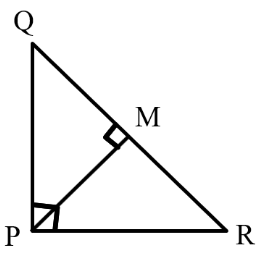Suppose that, in the above figure, $\angle MPR=x$.

Then, for the triangle $\Delta MPR$, we have

$\angle MRP={{180}^{\circ }}-{{90}^{\circ }}-x={{90}^{\circ }}-x$

Again, for the triangle $\Delta MPQ$, we have

$\angle MQP={{180}^{\circ }}-{{90}^{\circ }}-\left( {{90}^{\circ }}-x \right)=x$

Therefore, for both triangles $\Delta QMP$ and $\Delta PMR$, we obtain

$\angle MPQ=\angle MRP$,

$\angle PMQ=\angle RMP$, and

$\angle MQP=\angle MPR$.

Thus,$\Delta QMP\sim \Delta PMR$  [According to the $AAA$ similarity property]

So, $\frac{QM}{PM}=\frac{MP}{MR}$ and

hence, $P{{M}^{2}}=QM\times MR$.

3. In the following figure, $\mathbf{ABD}$ is a triangle right angled at $\mathbf{A}$ and $\mathbf{AC}\bot \mathbf{BD}$. Prove the following results.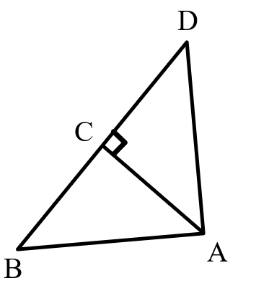(i)  $\mathbf{A}{{\mathbf{B}}^{\mathbf{2}}}=\mathbf{BC}\times \mathbf{BD}$

Ans: For the triangles $\Delta ADB$ and $\Delta ACB$, we have

$\angle DAB=\angle ACB$ [since both the angles are of ${{90}^{\circ }}$]

$\angle ABD=\angle CBA$ [common angles of the triangles]

Therefore, $\Delta ADB\sim \Delta CAB$ [According to the $AA$ similarity property]

So, $\frac{AB}{CB}=\frac{BD}{AB}$ and

hence, $A{{B}^{2}}=CB\times BD$.

(ii) $\mathbf{A}{{\mathbf{C}}^{\mathbf{2}}}=\mathbf{BC}\times \mathbf{DC}$

Ans: First suppose that, that $\angle CAB=x$.

Then, from the triangle $\Delta CBA$, we have

$\angle CBA={{180}^{\circ }}-{{90}^{\circ }}-x={{90}^{\circ }}-x$.

Again, from the triangle $\Delta CAD$, we have

$\angle CAD={{180}^{\circ }}-{{90}^{\circ }}-\left( {{90}^{\circ }}-x \right)=x$

Now, for both the triangles $\Delta CBA$ and $\Delta CAD$, we obtain

$\angle CBA=\angle CAD$,

$\angle CAB=\angle CDA$, and

$\angle ACB=\angle DCA$  [since, both the angles are of ${{90}^{\circ }}$]

Therefore, $\Delta CBA\sim \Delta CAD$ [According to the $AAA$ similarity property]

So, $\frac{AC}{DC}=\frac{BC}{AC}$ and

hence, $A{{C}^{2}}=DC\times BC$

(iii) $\mathbf{A}{{\mathbf{D}}^{\mathbf{2}}}=\mathbf{BD}\times \mathbf{CD}$

Ans: From the triangles $\Delta DCA$ and $\Delta DAB$, we obtain

$\angle DCA=\angle DAB$ [both the angles are of ${{90}^{\circ }}$]

$\angle CDA=\angle ADB$ [common angle of the triangles]

Thus, $\Delta DCA\sim \Delta DAB$ [by the $AA$ similarity property]

So, $\frac{DC}{DA}=\frac{DA}{DB}$ and

hence, $A{{D}^{2}}=BD\times CD$

4. $\mathbf{ABC}$ is an isosceles triangle right angled at $\mathbf{C}$. Prove that $\mathbf{A}{{\mathbf{B}}^{\mathbf{2}}}=\mathbf{2A}{{\mathbf{C}}^{\mathbf{2}}}$.

Ans: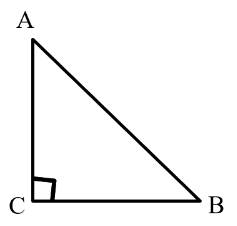We are provided that, $\Delta ABC$ is an isosceles triangle, and so,

$AC=CB$. Also, the angle $\angle C={{90}^{\circ }}$.

Now, by the Pythagorean theorem for the triangle $\Delta ABC$, gives

$A{{C}^{2}}+C{{B}^{2}}=A{{B}^{2}}$

$\Rightarrow A{{C}^{2}}+A{{C}^{2}}=A{{B}^{2}}$ [since $AC=CB$]

$\Rightarrow 2A{{C}^{2}}=A{{B}^{2}}$

5. $\mathbf{ABC}$ is an isosceles triangle with $\mathbf{AC}=\mathbf{BC}$. If $\mathbf{A}{{\mathbf{B}}^{\mathbf{2}}}=\mathbf{2A}{{\mathbf{C}}^{\mathbf{2}}}$, prove that $\mathbf{ABC}$ is a right triangle.

Ans: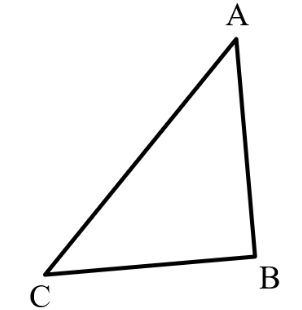We are provided that,

$A{{B}^{2}}=2A{{C}^{2}}$

Therefore, $A{{B}^{2}}=A{{C}^{2}}+A{{C}^{2}}$

$\Rightarrow A{{C}^{2}}+B{{C}^{2}}=A{{B}^{2}}$ [since it is given that $AC=BC$]

Thus, the triangle holds the Pythagorean theorem.

Hence, the provided triangle is a right triangle.

6. $\mathbf{ABC}$ is an equilateral triangle of side $\mathbf{2a}$. Find each of its altitudes.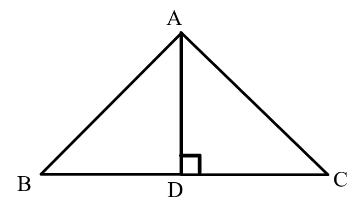Ans: Suppose that, the altitude in the provided equilateral triangle $\Delta ABC$ is $AD$. Now, recall that, altitude of an equilateral triangle bisects the opposite side.

Therefore, $BD=DC=a$.

For the triangle $\Delta ADB$, we have

$\angle ADB={{90}^{\circ }}$.

So, using the Pythagorean theorem, gives

$A{{D}^{2}}=D{{B}^{2}}+A{{B}^{2}}$

$\Rightarrow A{{D}^{2}}+{{a}^{2}}={{\left( 2a \right)}^{2}}$

$\Rightarrow A{{D}^{2}}+{{a}^{2}}=4{{a}^{2}}$

$\Rightarrow A{{D}^{2}}=3{{a}^{2}}$

$\Rightarrow AD=a\sqrt{3}$.

Again, since, in an equilateral triangle, all the altitudes are of equal length, so the length of each altitude is $a\sqrt{3}$ units.

7. Prove that the sum of the squares of the sides of rhombus is equal to the sum of the squares of its diagonals.

Ans: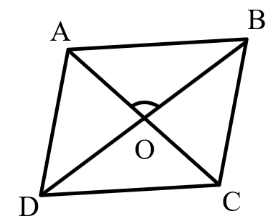Recall that, the diagonals of a rhombus intersect each other at ${{90}^{\circ }}$.

Therefore, using Pythagorean theorem for the triangles $\Delta AOB$, $\Delta BOC$, $\Delta COD$, and $\Delta AOD$, we get

$A{{B}^{2}}=A{{O}^{2}}+O{{B}^{2}}$                           …… (i)

$B{{C}^{2}}=B{{O}^{2}}+O{{C}^{2}}$                           …… (ii)

$C{{D}^{2}}=C{{O}^{2}}+O{{D}^{2}}$                           …… (iii)

$A{{D}^{2}}=A{{O}^{2}}+O{{D}^{2}}$                           …… (iv)

Add the equations (i), (ii), (iii) and (iv) together. Then, it yields

$A{{B}^{2}}+B{{C}^{2}}+C{{D}^{2}}+A{{D}^{2}}=2\left( A{{O}^{2}}+O{{B}^{2}}+O{{C}^{2}}+O{{D}^{2}} \right)$

$=2\left[ {{\left( \frac{AC}{2} \right)}^{2}}+{{\left( \frac{BD}{2} \right)}^{2}}+{{\left( \frac{AC}{2} \right)}^{2}}+{{\left( \frac{BD}{2} \right)}^{2}} \right]$ [since the diagonal bisect one another]

$=2\left[ \frac{{{\left( AC \right)}^{2}}}{2}+\frac{{{\left( BD \right)}^{2}}}{2} \right]$

Thus, $A{{B}^{2}}+B{{C}^{2}}+C{{D}^{2}}+A{{D}^{2}}=A{{C}^{2}}+B{{D}^{2}}$.

Hence, the required result is proved.

8. In the following figure, $\mathbf{O}$ is a point in the interior of a triangle $\mathbf{ABC}$, $\mathbf{OD}\bot \mathbf{BC}$, $\mathbf{OE}\bot \mathbf{AC}$ and $\mathbf{OF}\bot \mathbf{AB}$. Prove the following results.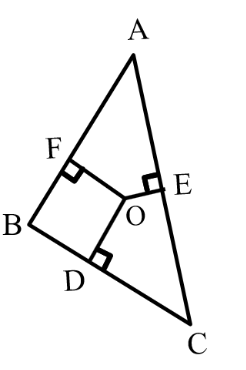(i) $\mathbf{O}{{\mathbf{A}}^{\mathbf{2}}}\mathbf{+ O}{{\mathbf{B}}^{\mathbf{2}}}\mathbf{+ O}{{\mathbf{C}}^{\mathbf{2}}}-\mathbf{O}{{\mathbf{D}}^{\mathbf{2}}}-\mathbf{O}{{\mathbf{E}}^{\mathbf{2}}}-\mathbf{O}{{\mathbf{F}}^{\mathbf{2}}}\mathbf{=A}{{\mathbf{F}}^{\mathbf{2}}}\mathbf{+ B}{{\mathbf{D}}^{\mathbf{2}}}\mathbf{+ C}{{\mathbf{E}}^{\mathbf{2}}}$

Ans: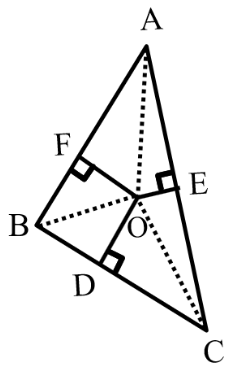Using the Pythagorean theorem for the triangles $\Delta AOF$, $\Delta BOD$, and $\Delta COE$, it yields

$O{{A}^{2}}=O{{F}^{2}}+A{{F}^{2}}$                                                 …… (i)

$O{{B}^{2}}=O{{D}^{2}}+B{{D}^{2}}$,                                               …… (ii)

$O{{C}^{2}}=O{{E}^{2}}+E{{C}^{2}}$                                                 …… (iii)

Now, add the equations (i), (ii) and (iii) together. Then we get

$O{{A}^{2}}+O{{B}^{2}}+O{{C}^{2}}=O{{F}^{2}}+A{{F}^{2}}+O{{D}^{2}}+B{{D}^{2}}+O{{E}^{2}}+E{{C}^{2}}$

Hence,

$O{{A}^{2}}+O{{B}^{2}}+O{{C}^{2}}-O{{D}^{2}}-O{{E}^{2}}-O{{F}^{2}}=A{{F}^{2}}+B{{D}^{2}}+E{{C}^{2}}$.

(ii) $\mathbf{A}{{\mathbf{F}}^{\mathbf{2}}}\mathbf{+ B}{{\mathbf{D}}^{\mathbf{2}}}\mathbf{+ C}{{\mathbf{E}}^{\mathbf{2}}}\mathbf{=A}{{\mathbf{E}}^{\mathbf{2}}}\mathbf{+ C}{{\mathbf{D}}^{\mathbf{2}}}\mathbf{+ B}{{\mathbf{F}}^{\mathbf{2}}}$

Ans: Referring from the above subpart, we have

$O{{A}^{2}}+O{{B}^{2}}+O{{C}^{2}}-O{{D}^{2}}-O{{E}^{2}}-O{{F}^{2}}=A{{F}^{2}}+B{{D}^{2}}+E{{C}^{2}}$

$\Rightarrow A{{F}^{2}}+B{{D}^{2}}+E{{C}^{2}}=\left( O{{A}^{2}}-O{{E}^{2}} \right)+\left( O{{C}^{2}}-O{{D}^{2}} \right)+\left( O{{B}^{2}}-O{{F}^{2}} \right)$

Thus, $A{{F}^{2}}+B{{D}^{2}}+C{{E}^{2}}=A{{E}^{2}}+C{{D}^{2}}+B{{F}^{2}}$

9. a ladder $\mathbf{10}$ m long reaches a window $\mathbf{8}$ m above the ground. Find the distance of the foot of the ladder from the base of the wall.

Ans: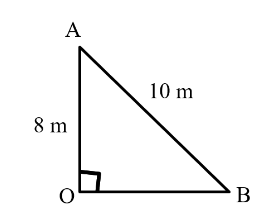In the above figure, suppose that $OA$ represents the wall and $AB$ represents the ladder. So, applying the Pythagorean theorem, we get

$A{{B}^{2}}=O{{A}^{2}}+B{{O}^{2}}$

$\Rightarrow {{\left( 10 \right)}^{2}}={{8}^{2}}+{{\left( OB \right)}^{2}}$

$\Rightarrow 100=64+{{\left( OB \right)}^{2}}$

$\Rightarrow {{\left( OB \right)}^{2}}=36$

$\Rightarrow OB=6$ m

Hence, the distance of the foot of the ladder from the base of the wall is $6$ m.

10. A guy wire attached to a vertical pole of height $\mathbf{18}$ m is $\mathbf{24}$ m long and has a stake attached to the other end. How far from the base of the pole should the stake be driven so that the wire will be taut?

Ans: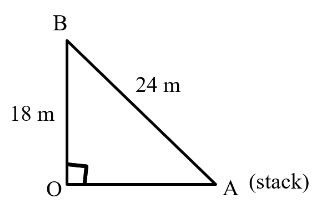Suppose that, in the above figure, $OB$ represents the pole and $AB$ represents the wire. Then, applying the Pythagorean theorem, we obtain

$A{{B}^{2}}=O{{B}^{2}}+O{{A}^{2}}$

$\Rightarrow {{\left( 24 \right)}^{2}}={{\left( 18 \right)}^{2}}+{{\left( OA \right)}^{2}}$

$\Rightarrow {{\left( OA \right)}^{2}}=576-324=252$

$\Rightarrow OA=\sqrt{252}=\sqrt{6\times 6\times 7}=6\sqrt{7}$ m

Hence, the stack should be driven $6\sqrt{7}$ m from the base of pole.

11. An aeroplane leaves an airport and flies due north at a speed of $\mathbf{1},\mathbf{000}$ km per hour. At the same time, another aeroplane leaves the same airport and flies due west at a speed of $\mathbf{1},\mathbf{200}$ km per hour. How far apart will be the two planes after $\mathbf{1}\frac{\mathbf{1}}{\mathbf{2}}$ hours?

Ans: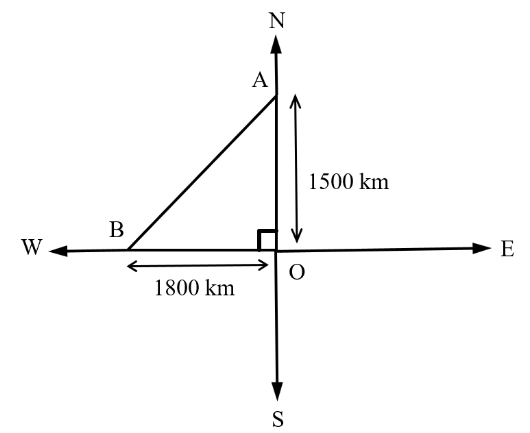The distance that the plane travelled towards the north in $1\frac{1}{2}$ hours

$=1000\times 1\frac{1}{2}=1500$ km

In a similar way, the distance that the plane travelled towards the west in $1\frac{1}{2}$ hours $=1200\times 1\frac{1}{2}=1800$ km.

Suppose that distances $OA$ and $OB$ respectively are the distances discussed above. Then, by the Pythagorean theorem,

the distance between the two planes after $1\frac{1}{2}$ hours, $AB=\sqrt{O{{A}^{2}}+O{{B}^{2}}}$

$=\left( \sqrt{{{1500}^{2}}+{{1800}^{2}}} \right)$

$=\sqrt{2250000+3240000}$

$=\sqrt{5490000}$

$=\sqrt{9\times 610000}$

$=300\sqrt{61}$ km

Hence, after $1\frac{1}{2}$ hours, the planes will be $300\sqrt{61}$ km far from one another.

12. Two poles of heights $\mathbf{6}$ m and $\mathbf{11}$ m stand on a plane ground. If the distance between the feet of the poles is $\mathbf{12}$ m, find the distance between their tops.

Ans: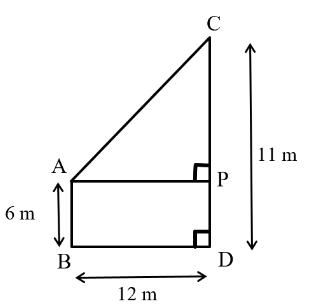Suppose that, $CD=11$m and $AB=6$m are the poles.

So, $CP=11-6=5$ m.

Then, by observing the above figure, we get $AP=12$ m.

Now, using the Pythagorean theorem for the triangle $\Delta APC$, gives

$A{{P}^{2}}+P{{C}^{2}}=A{{C}^{2}}$

$\Rightarrow {{12}^{2}}+{{5}^{2}}={{\left( AC \right)}^{2}}$

$\Rightarrow A{{C}^{2}}=144+25=169$ .

$\Rightarrow AC=13$ m

13. $\mathbf{D}$ and $\mathbf{E}$ are points on the sides $\mathbf{CD}$ and $\mathbf{CB}$ respectively of a triangle $\mathbf{ABC}$ right angled at $\mathbf{C}$. Prove that $\mathbf{A}{{\mathbf{E}}^{\mathbf{2}}}+\mathbf{B}{{\mathbf{D}}^{\mathbf{2}}}=\mathbf{A}{{\mathbf{B}}^{\mathbf{2}}}+\mathbf{D}{{\mathbf{E}}^{\mathbf{2}}}$.

Ans: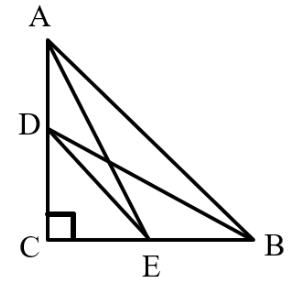Using the Pythagorean theorem for the triangles $\Delta ACE$,  and $\Delta BCD$, yields,

$A{{C}^{2}}+C{{E}^{2}}=A{{E}^{2}}$                                              …… (i)

$B{{C}^{2}}+C{{D}^{2}}=B{{D}^{2}}$                                              …… (ii)

Then, the equations (i) and (ii) together implies that

$A{{C}^{2}}+C{{E}^{2}}+B{{C}^{2}}+C{{D}^{2}}=A{{E}^{2}}+B{{D}^{2}}$              …… (iii)

Again, using the Pythagorean theorem for the triangle $\Delta CDE$ and $\Delta ABC$, we have

$D{{E}^{2}}=C{{D}^{2}}+C{{E}^{2}}$                                              …… (iv)

and $A{{B}^{2}}=A{{C}^{2}}+C{{B}^{2}}$                                        …… (v)

Adding the equations (iv) and (v), it gives

$D{{E}^{2}}+A{{B}^{2}}=C{{D}^{2}}+C{{E}^{2}}+A{{C}^{2}}+C{{B}^{2}}$

$\Rightarrow D{{E}^{2}}+A{{B}^{2}}=A{{E}^{2}}+B{{D}^{2}}$ [using the equation (iii)]

Hence, the required result is proved.

14. The perpendicular from $\mathbf{A}$ on side $\mathbf{BC}$ of a $\mathbf{\Delta ABC}$ intersect $\mathbf{BC}$ at $\mathbf{D}$ such that $\mathbf{DB}=\mathbf{3CD}$. Prove that $\mathbf{2A}{{\mathbf{B}}^{\mathbf{2}}}=\mathbf{2A}{{\mathbf{C}}^{\mathbf{2}}}+\mathbf{B}{{\mathbf{C}}^{\mathbf{2}}}$.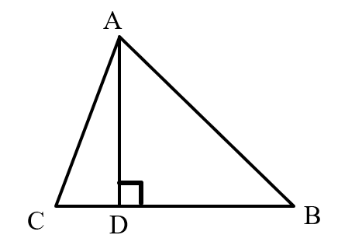Ans: Using the Pythagorean theorem in the triangle $\Delta ACD$, it gives

$A{{C}^{2}}=A{{D}^{2}}+D{{C}^{2}}$

$\Rightarrow A{{D}^{2}}=A{{C}^{2}}-D{{C}^{2}}$                            …… (i)

Again, using the Pythagorean theorem in the triangle $\Delta ABD$, gives

$A{{B}^{2}}=A{{D}^{2}}+D{{B}^{2}}$

$\Rightarrow A{{D}^{2}}=A{{B}^{2}}-D{{B}^{2}}$                             …… (ii)

Then, the equations (i) and (ii) together implies that

$A{{C}^{2}}-D{{C}^{2}}=A{{B}^{2}}-D{{B}^{2}}$                          …… (iii)

Now, we are provided that, $3DC=DB$.

So, $DC=\frac{BC}{4}$,                                           …… (iv)

$DB=\frac{3BC}{4}$ .                                              …… (v)

Substituting the values of $DC$ and $DB$ from the equations (iv) and (v) into the equation (iii), gives

$A{{C}^{2}}-{{\left( \frac{BC}{4} \right)}^{2}}=A{{B}^{2}}-{{\left( \frac{3BC}{4} \right)}^{2}}$

$\Rightarrow A{{C}^{2}}-\frac{B{{C}^{2}}}{16}=AB-\frac{9B{{C}^{2}}}{16}$

$\Rightarrow 16A{{C}^{2}}-B{{C}^{2}}=16A{{B}^{2}}-9B{{C}^{2}}$

$\Rightarrow 16A{{B}^{2}}-16A{{C}^{2}}=8B{{C}^{2}}$

$\Rightarrow 2A{{B}^{2}}=2A{{C}^{2}}+B{{C}^{2}}$

15. In an equilateral triangle $\mathbf{ABC}$, $\mathbf{D}$ is a point on side $\mathbf{BC}$ such that $\mathbf{BD}=\frac{\mathbf{1}}{\mathbf{3}}\mathbf{BC}$. Prove that $\mathbf{9A}{{\mathbf{D}}^{\mathbf{2}}}=\mathbf{7A}{{\mathbf{B}}^{\mathbf{2}}}$.

Ans: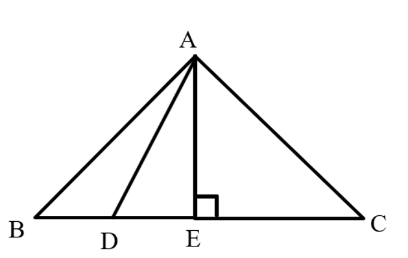Suppose that, the length of the side of the given equilateral triangle $\Delta ABC$ is $a$ units, and $AE$ is its altitude.

Then, $BE=EC=\frac{BC}{2}=\frac{a}{2}$,

$AE=\frac{a\sqrt{3}}{2}$.

Also, we are provided that $BD=\frac{1}{3}BC$

Therefore, $BD=\frac{a}{3}$.

So, $DE=BE-BD=\frac{a}{2}-\frac{a}{3}=\frac{a}{6}$.

Now, using Pythagorean theorem for the triangle $\Delta ADE$, gives

$A{{D}^{2}}=A{{E}^{2}}+D{{E}^{2}}$

$\Rightarrow A{{D}^{2}}={{\left( \frac{a\sqrt{3}}{2} \right)}^{2}}+{{\left( \frac{a}{6} \right)}^{2}}$

$=\frac{3{{a}^{2}}}{4}+\frac{{{a}^{2}}}{36}$

$=\frac{28{{a}^{2}}}{36}$

$=\frac{7}{9}A{{B}^{2}}$

Hence, $9A{{D}^{2}}=7A{{B}^{2}}$

16. In an equilateral triangle, prove that three times the square of one side is equal to four times the square of one of its altitudes.

Ans: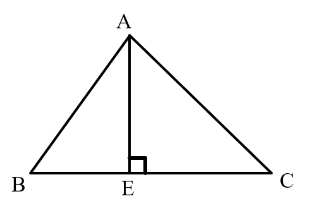Suppose that, length of the side of the given equilateral triangle $\Delta ABC$ is $a$, and $AE$ is its altitude.

Therefore, $BE=EC=\frac{BC}{2}=\frac{a}{2}$.

Now, using the Pythagorean theorem for the triangle $\Delta ABE$, gives

$A{{B}^{2}}=A{{E}^{2}}+B{{E}^{2}}$

$\Rightarrow {{a}^{2}}=A{{E}^{2}}+{{\left( \frac{a}{2} \right)}^{2}}$

$\Rightarrow A{{E}^{2}}={{a}^{2}}-\frac{{{a}^{2}}}{4}$

$\Rightarrow A{{E}^{2}}=\frac{3{{a}^{2}}}{4}$

Hence, $4\times$ (square of altitude) $=3\times$ (square of one side).

17. Tick the correct answer and justify: In $\Delta \,\mathbf{ABC}$, $\mathbf{AB}=\mathbf{6}\sqrt{\mathbf{3}}$ cm, $\mathbf{AC}=\mathbf{12}$ cm and $\mathbf{BC}=\mathbf{6}$ cm.

The angle $\mathbf{B}$ is:

(A) $\mathbf{12}{{\mathbf{0}}^{\mathbf{o}}}$

(B) $\mathbf{6}{{\mathbf{0}}^{\mathbf{o}}}$

(C) $\mathbf{9}{{\mathbf{0}}^{\mathbf{o}}}$

(D) $\mathbf{4}{{\mathbf{5}}^{\mathbf{o}}}$

Ans: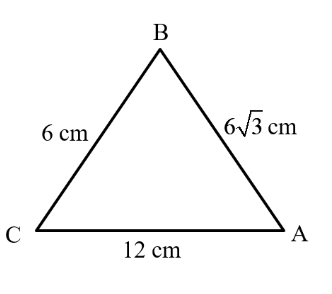We are provided that, $AB=6\sqrt{3}$ cm, $AC=12$ cm, and $BC=6$ cm.

We can notice that, $A{{B}^{2}}=108$

$A{{C}^{2\text{ }}}\text{= }144$, $B{{C}^{2}}=36$.

Therefore,

$A{{B}^{2}}+B{{C}^{2}}=108+36=144=A{{C}^{2}}$.

Thus, the triangle $\Delta ABC$ satisfies the Pythagorean theorem.

Hence, the provided triangle is a right triangle.

So, $\angle B={{90}^{\circ }}$.

Hence, option (c) is the correct answer.

## CBSE Class 10 Maths Chapter 6 Exercise 6.5 Solutions Free PDF

Now you can get answers to every question in Class 10 Maths Chapter 6.5 in PDF format on the official website of Vedantu. These PDFs can be downloaded anytime anywhere on your device or printed out, and then you can access them without an internet connection. The NCERT Class 10 Ex 6.5 PDF is the easiest and quickest way to revise all the formulas and tips for the Pythagorean theorem and its applications.

### Exercise 6.5 Class 10 Question 1

The first question of Ex 6.5 Class 10 has measurements of the sides of 4 different triangles. For each of them, you need to apply the Pythagoras theorem to determine if they are right-angled triangles or not. You also need to calculate the hypotenuse of the right-angle triangles.

### Exercise 6.5 Class 10 Question 2

A pictorial diagram of a triangle PQR (right-angled at P) is presented to you in this question of 6.5 Exercise Class 10. A perpendicular PM is dropped from P to the side QR, and you need to prove them PM² = MQ * MR. This can be done by using the AAA similarity criterion and concept of Pythagoras theorem.

### Exercise 6.5 Class 10 Question 3

In the 3rd question of maths Class 10 6.5, a triangle ABC is given along with 3 statements regarding its sides. You need to prove those statements by using AA and AAA similarity criteria. If you prove the first two statements, the third statement gets proved automatically.

### Exercise 6.5 Class 10 Question 4

Maths Class 10 Ex 6.5 question 4 has an isosceles triangle ABC which is right-angled at C. You need to prove that AB² = 2*AC² and you can prove this by the simplest form of application of the Pythagoras theorem.

### Exercise 6.5 Class 10 Question 5

This question again has an isosceles triangle where AC = BC. You need to prove that it is a right-angle triangle by applying the converse of the Pythagoras theorem. You could use the knowledge gained in class 10 about triangles to solve this question.

### Exercise 6.5 Class 10 Question 6

This question presents a triangle ABC in a diagram that has sides = 2a. Students have to find the length of each of the altitudes. You can do this by drawing a perpendicular AD from A on side BC and apply the theorem that the altitude of an equilateral triangle bisects the opposite sides. Along with this feature of an equilateral triangle, you also need to use the Pythagorean theorem to answer this question.

### Exercise 6.5 Class 10 Question 7

Here you need to prove that in a rhombus the sum of the square of its sides is equal to the sum of squares of its diagonals. This needs the application of your knowledge in equilateral triangles and the Pythagoras theorem to prove this statement.

### Exercise 6.5 Class 10 Question 8

Question 8 in exercise 6.5 Class 10 Maths requires basic concepts of triangles to prove two relations given. Approach this problem in a step-by-step manner, and you can easily do it.

### Exercise 6.5 Class 10 Question 9

It is a practical problem involving a ladder which is put against a window, and you need to find the distance of the foot of the ladder from the base of the wall. This question tests your aptitude in trigonometric derivatives, and you need to apply the Pythagoras theorem to calculate the height and distance.

### Exercise 6.5 Class 10 Question 10

You can solve this question if your core knowledge of Pythagoras theorem is strong. It is a practical problem that is a bit tricky; hence just knowing the theorem would not suffice. With our diagrammatic approach to the question, you should be able to understand the derivation clearly.

### Exercise 6.5 Class 10 Question 11

The central idea of the 10th question is combining your knowledge of trigonometry with the Pythagoras theorem. It is again a practical problem where two aeroplanes’ speed, flying North and West is given, and you need to find the distance between the two planes after a certain time lapse.

### Exercise 6.5 Class 10 Question 12

This is another problem that contains the application of both Pythagoras theorem and trigonometry concepts. Your ease in such problems will develop as you keep doing such problems and building your foundation in both these aspects of mathematics.

### Exercise 6.5 Class 10 Question 13

In a right-angled triangle, ABC, right-angled at C, two points D and E are taken on sides AC and BC respectively. One needs to prove equations involving the interrelation of triangles and the Pythagoras theorem.

### Exercise 6.5 Class 10 Question 14

This question needs an elaborate implementation of the Pythagoras theorem and is a lengthy problem where you are given the figure of a triangle ABC. A perpendicular is drawn from point A on BC which intersects the side at D, and you need to prove a statement involving these sides of the triangle.

### Exercise 6.5 Class 10 Question 15

Question 15 of this exercise is based on an equilateral triangle, and you are given various features of that triangle. It is one of the complicated problems, but you can easily understand it once you go through our solutions. You would need to draw an imaginary altitude from A to BC. Then you can apply the property of the altitude of an equilateral triangle that bisects the opposite side to derive the equation given.

### Exercise 6.5 Class 10 Question 16

This question requires you to prove some facts about an equilateral triangle. You need to prove that in an equilateral triangle if you multiply the square of one side by 3 then it is equal to 4 times the square of one of its altitudes.

### Exercise 6.5 Class 10 Question 17

The last question is a multiple-choice question where you need to find out one specific angle of a triangle. You are given the length of the sides of the triangle and apply Pythogaros triplets to find the solution.

### Key Features of NCERT Solutions for Class 10 Maths Chapter 6 Exercise 6.5

The NCERT Solutions Class 10 Maths Ex 6.5 is prepared by the expert team of Vedantu keeping in mind the understanding level of class 10 students. Hence students will find it easy to go through them. Some of the main benefits of these solutions are:

• The solutions will clarify your doubts at the root level so that you can successfully attempt similar problems independently.

• The answers to each question are there, and nothing is left out.

• The mathematicians at Vedantu have taken care of the CBSE pattern and NCERT guidelines to answer these questions so you can be sure of scoring good marks with our solutions.

• Having the solution in PDF format is very convenient for quick revisions.

## FAQs on NCERT Solutions for Class 10 Maths Chapter 6 - Exercise

1. What is the Main Topic of Exercise 6.5 of Class 10th Maths, Chapter 6?

Ans: Exercise 6.5 of chapter 6 of class 10th maths is based on theorems involving right-angled triangles. The basis of the problems is the Pythagoras theorem along with many concepts you have learned about triangles in previous chapters. There are questions based on equilateral triangles, isosceles triangles, etc. for which you need to remember formulas or theorems revolving around these special triangles.

2. State the Pythagoras Theorem.

Ans: The Pythagoras theorem in mathematics denotes the fundamental relation between the three sides of a right-angle triangle. According to the Pythagoras theorem, in a right-angle triangle, the value of the square of the hypotenuse (this is the side opposite to the angel which is 90°) is equal to the summation of the squares of the other two sides so if ABC is a triangle where angle C is 90 degrees then:

AB² = BC² + AC².